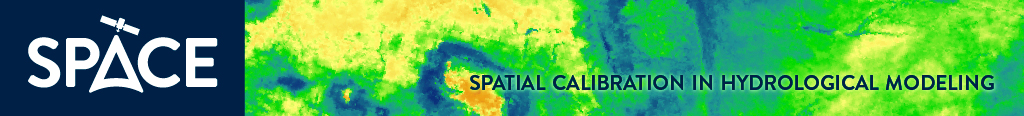To main > Tools and products

# Tools and products

## SPAtial EFficiency (SPAEF) – a new metric for pattern comparisonDeveloping new metrics for spatial pattern comparison of observed and simulated variables has been planned as major part of the first work package (WP1) of the SPACE project. This goal was achieved by developing SPAtial EFficiency metric (SPAEF) described below. For that first we have extensively investigated the current state-of-art spatial comparison metrics. We tested available metrics such as coefficient of variation, correlation coefficient, Goodman and Kruskal’s lambda (Goodman and Kruskal, 1954), Mapcurves (Hargrove et al., 2006), agreement coefficient (Ji and Gallo, 2006), FSS, Theil’s Uncertainty, EOF and Cramér’s V (Cramér, 1946; Koch et al., 2015; Rees, 2008) in calibration of a distributed hydrologic model. However, after many calibration attempts we found them inadequate due to the unexpected resultant visual patterns resulted in high correlation but too low standard deviation or highly separate groups. Therefore, we introduced a brand new multi-component SPAEF metric in Demirel et al. (2018) which showed the utility of SPAEF in an ensemble model calibration case. For further comparison of the SPAEF with other metrics and particularly analysing each component separately in a calibration framework please refer to the subsequent study by Koch et al. (2018). Illustrative examples and source codes are available in Python 2.7 and Matlab (Demirel et al., 2017).

The SPAEF has an optimal value of 1 and defined as:

 SPAEF=1-[(A-1)2+(B-1)2+(C-1)2]1/2 A=Correlation Coefficient (O,S) B=COV(O)/ COV(S) C=Histogram Match (O,S)

where A is the Pearson’s correlation coefficient between observed AET map (O) and simulated AET map (S) for a particular month, B is the fraction of coefficient of variations representing spatial variability and C is the percentage of histogram intersection (Swain and Ballard, 1991). The C is calculated for a given histogram O of the observed AET map (O) and the histogram S of the model simulated AET map (S), each one containing n bins i.e. herein 100 bins. It is important to note that the raster maps are normalized to a mean of 0 and a standard deviation equal to 1 (zscore) to avoid the effect of different units.

References:

Cramér, H.: Mathematical Methods of Statistics, Princeton University Press., 1946.

Demirel, M. C., Koch, J. and Stisen, S.: SPAEF: SPAtial EFficiency, Researchgate, doi:10.13140/RG.2.2.18400.58884, 2017.

Demirel, M. C., Mai, J., Mendiguren, G., Koch, J., Samaniego, L. and Stisen, S.: Combining satellite data and appropriate objective functions for improved spatial pattern performance of a distributed hydrologic model, Hydrol. Earth Syst. Sci., 22(2), 1299–1315, doi:10.5194/hess-22-1299-2018, 2018.

Goodman, L. A. and Kruskal, W. H.: Measures of Association for Cross Classifications*, J. Am. Stat. Assoc., 49(268), 732–764, doi:10.1080/01621459.1954.10501231, 1954.

Hargrove, W. W., Hoffman, F. M. and Hessburg, P. F.: Mapcurves: a quantitative method for comparing categorical maps, J. Geogr. Syst., 8(2), 187–208, doi:10.1007/s10109-006-0025-x, 2006.

Ji, L. and Gallo, K.: An agreement coefficient for image comparison, Photogramm. Eng. Remote Sensing, 72(7), 823–833 [online] Available from: http://pubs.er.usgs.gov/publication/70028506, 2006.

Koch, J., Jensen, K. H. and Stisen, S.: Toward a true spatial model evaluation in distributed hydrological modeling: Kappa statistics, Fuzzy theory, and EOF-analysis benchmarked by the human perception and evaluated against a modeling case study, Water Resour. Res., 51(2), 1225–1246, doi:10.1002/2014WR016607, 2015.

Koch, J., Demirel, M.C., Stisen, S., (2018). "The SPAtial EFficiency metric (SPAEF): multiple-component evaluation of spatial patterns for optimization of hydrological models". Geosci. Model Dev. 11, 1873–1886.

Rees, W. G.: Comparing the spatial content of thematic maps, Int. J. Remote Sens., 29(13), 3833–3844, doi:10.1080/01431160701852088, 2008.

Swain, M. J. and Ballard, D. H.: Color indexing, Int. J. Comput. Vis., 7(1), 11–32, doi:10.1007/BF00130487, 1991.© SPACE Oester Voldgade 10, 1350 Copenhagen K Tlf.: +45 38142000 E-mail: